# Basics of simulating sawn timber strength with WoodSimulatR

library(WoodSimulatR)
library(magrittr)
library(ggplot2)
pander::panderOptions('knitr.auto.asis', FALSE);

# Introduction

The WoodSimulatR package provides functions for generating artificial datasets of sawn wood properties obtained by destructive and non-destructive testing.

An existing dataset containing some of these properties can be enriched by adding simulated values for the missing properties.

## Aim of this document

This document aims to provide an overview of the capabilities of the WoodSimulatR package.

On the one hand, we will simulate a dataset with varying parameters, highlighting both the capabilities of the WoodSimulatR functions and the direction where it should go.

On the other hand, we will illustrate the capabilities of WoodSimulatR with respect to simulating grade determining properties for a dataset with different pre-existing variables.

# Simulate a whole dataset

## Preliminaries

As a quick summary of each dataset, we will show mean and CoV for all variables, split by country and subsample, and we will show the matrix of correlations.

For this, we define the following function:

summ_fun <- function(ds) {
grp <- intersect(grp, names(ds));
v <- setdiff(names(ds), grp);

r <- cor(ds[v]);

ds <- tibble::add_column(ds, n = 1);
v <- c('n', v);
ds <- tidyr::gather(ds, 'property', 'value', !!! rlang::syms(v));
ds <- dplyr::mutate(
ds,
property = factor(
property,
levels=v,
labels=ifelse(v=='n', v, paste0(v, '_mean')),
ordered = TRUE
)
);

grp <- c(grp, 'property');
ds <- dplyr::group_by(ds, !!! rlang::syms(grp));

summ <- dplyr::summarise(
ds,
res = if (property == 'n') sprintf('%.0f', sum(value)) else
sprintf(
if(property %in% c('f_mean', 'ip_f_mean')) '%.1f (%.0f)' else '%.0f (%.0f)',
mean(value), 100*sd(value)/mean(value)),
.groups = 'drop_last'
);
pander::pander(
split.tables = Inf
);

pander::pander(r)

invisible(summ);
}

compare_with_def <- function(ds, ssd, target = c('mean', 'cov')) {
target <- match.arg(target);

ds <- dplyr::group_by(ds, country);
summ <- dplyr::summarise(
ds,
f_mean.ach = mean(f),
f_cov.ach = sd(f) / f_mean.ach,
E_mean.ach = mean(E),
E_cov.ach = sd(E) / E_mean.ach,
rho_mean.ach = mean(rho),
rho_cov.ach = sd(rho) / rho_mean.ach,
.groups = 'drop_last'
);

stopifnot(!anyDuplicated(ssd$country)); summ <- dplyr::left_join( summ, dplyr::select( dplyr::mutate(ssd, f_cov = f_sd / f_mean, E_cov = E_sd / E_mean, rho_cov = rho_sd / rho_mean), country, f_mean, f_cov, E_mean, E_cov, rho_mean, rho_cov ), by = 'country' ); summ <- tidyr::pivot_longer( summ, -country, names_to = c('gdpname', '.value'), names_sep = '_' ); summ <- dplyr::mutate( summ, gdpname = factor(gdpname, levels = c('f', 'E', 'rho'), ordered = TRUE) ); if (target == 'mean') { ggplot(data = summ, aes(mean.ach, mean)) + geom_abline(slope = 1, intercept = 0) + geom_text(aes(label = country)) + geom_point(alpha = 0.5) + facet_wrap(vars(gdpname), scales = 'free') + theme(axis.text.x = element_text(angle = 90)); } else { ggplot(data = summ, aes(cov.ach, cov)) + geom_abline(slope = 1, intercept = 0) + geom_text(aes(label = country)) + geom_point(alpha = 0.5) + facet_wrap(vars(gdpname), scales = 'free') + theme(axis.text.x = element_text(angle = 90)); } } ## Default dataset The main function for dataset simulation is simulate_dataset(). It can be called without any further arguments to yield a “default” dataset. For reproducibility, we will call it with the extra argument random_seed = 12345. This means that we will always generate the same random numbers. dataset_0 <- simulate_dataset(random_seed = 2345); #> Warning in RNGkind("Mersenne-Twister", "Inversion", "Rounding"): non-uniform #> 'Rounding' sampler used summ_fun(dataset_0); country subsample loadtype n f_mean E_mean rho_mean E_dyn_u_mean ip_f_mean E_dyn_mean ip_rho_mean C1 C1 t 1250 26.1 (39) 10858 (19) 436 (11) 10334 (19) 25.0 (33) 11704 (18) 452 (11) C2 C2 t 1250 26.8 (37) 11573 (20) 423 (10) 10829 (19) 28.2 (33) 12160 (19) 442 (9) C3 C3 t 1250 31.4 (40) 10356 (22) 443 (11) 10044 (20) 24.2 (37) 11367 (20) 454 (10) C4 C4 t 1250 25.4 (42) 10822 (22) 405 (10) 10122 (20) 26.0 (37) 11357 (20) 425 (10) f E rho E_dyn_u ip_f E_dyn ip_rho f 1 0.6938 0.332 0.6209 0.7269 0.6518 0.3103 E 0.6938 1 0.5475 0.9033 0.9099 0.9487 0.5694 rho 0.332 0.5475 1 0.5953 0.3754 0.6661 0.9417 E_dyn_u 0.6209 0.9033 0.5953 1 0.8512 0.9416 0.6193 ip_f 0.7269 0.9099 0.3754 0.8512 1 0.883 0.3668 E_dyn 0.6518 0.9487 0.6661 0.9416 0.883 1 0.6984 ip_rho 0.3103 0.5694 0.9417 0.6193 0.3668 0.6984 1 The meaning of the properties in dataset_0 is as follows: • f: strength of the sawn timber in N/mm² as obtained by destructive testing (tensile strength or bending strength) • E: Modulus of elasticity in N/mm² as obtained by destructive testing in tension or in bending • rho (as from the Greek symbol $$\rho$$): density of a small clear sample cut from the sawn timber, in kg/m³ • E_dyn_u: dynamic modulus of the green sawn timber in N/mm² • ip_f: an “indicating property” (IP) for the strength f in N/mm², obtained non-destructively as a function of E_dyn, ip_rho and the total knot area ratio (tKAR) of a knot cluster of length 150mm (knot_tc – not included in the dataset). • IP for tension strength: $$ip_f = 11.98 + 0.003913 E_{dyn} - 0.04822 ip_\rho - 34.72 * knot_{tc}$$ • IP for bending strength: $$ip_f = 21.67 + 0.004302 E_{dyn} - 0.05366 * ip_\rho - 38.43 knot_{tc}$$ • E_dyn: dynamic modulus of the dry sawn timber in N/mm² • ip_rho: density of the whole board in kg/m³, calculated by weighing the board and dividing the weight by the product of the measured dimensions. All properties except E_dyn_u are to be taken as measured on the dry timber and corrected to a moisture content of 12%. ## Customising options The default dataset created above relies on the following assumptions: • It’s a dataset for tensile strength of spruce (Picea abies). • The correlations are taken from the “full” sample of Holzforschung Austria’s research project SiOSiP (“SImulation-based Optimisation of Sawn tImber Production”, 2014-2017) on Austrian spruce wood. • After log-transforming $$f$$, for all variables a normal distribution is assumed. • We create data for 5000 boards, split equally between four subsamples. • The means and standard deviations of $$f$$, $$E$$ and $$rho$$ are based on random values chosen from the range of available reference values. • The reference values for mean and standard deviation of transformed variables ($$f$$ in our case) are currently enforced exactly instead of statistically – this has to be improved yet. All of these assumptions can be modified more or less freely. ## Available subsample definitions (tension) get_subsample_definitions(loadtype = 't') %>% dplyr::select(-species, -loadtype) %>% dplyr::arrange(country) %>% pander::pander(split.table = Inf); project country share f_mean f_sd E_mean E_sd rho_mean rho_sd literature subsample siosip at 1 29.4 11.3 11912 2232 443 42.1 null at gradewood at 1 25.1 10.54 10100 2626 435 52.2 Ranta-Maunus et al. (2011) at_1 gradewood ch 1 27.8 12.23 10900 2616 439 52.68 Ranta-Maunus et al. (2011) ch gradewood de 1 32.6 12.06 12100 2541 451 49.61 Ranta-Maunus et al. (2011) de gradewood fi 1 33.2 11.29 11800 2242 445 44.5 Ranta-Maunus et al. (2011) fi null lv 1 30.4 11.55 11700 2808 466 51.26 Stapel et al. (2014) lv gradewood pl 1 28.2 10.72 11600 2668 452 54.24 Ranta-Maunus et al. (2011) pl gradewood ro 1 25.6 10.75 10000 2100 390 31.2 Ranta-Maunus et al. (2011) ro gradewood se 1 27.6 10.49 10200 2346 416 49.92 Ranta-Maunus et al. (2011) se gradewood si 1 34 14.96 12300 2706 442 39.78 Ranta-Maunus et al. (2011) si gradewood sk 1 27.2 10.88 10700 2140 408 36.72 Ranta-Maunus et al. (2011) sk gradewood ua 1 26.7 11.75 10300 2163 392 43.12 Ranta-Maunus et al. (2011) ua ## Available subsample definitions (bending) get_subsample_definitions(loadtype = 'be') %>% dplyr::select(-species, -loadtype) %>% dplyr::arrange(country) %>% pander::pander(split.table = Inf); project country share f_mean f_sd E_mean E_sd rho_mean rho_sd literature subsample siosip at 1 41.4 12.7 12294 2636 436 39.9 null at gradewood de 1 41.5 14.11 12100 3146 441 48.51 Ranta-Maunus et al. (2011) de gradewood fr 1 42.9 11.15 11900 2023 440 44 Ranta-Maunus et al. (2011) fr gradewood pl 1 38.5 11.94 11400 2280 440 48.4 Ranta-Maunus et al. (2011) pl gradewood ro 1 35.5 11.01 9600 1824 387 38.7 Ranta-Maunus et al. (2011) ro gradewood se 1 42.5 14.87 11300 2486 435 52.2 Ranta-Maunus et al. (2011) se gradewood se 1 44.8 13.44 12300 2706 435 52.2 Ranta-Maunus et al. (2011) se_1 gradewood si 1 43.7 13.11 12000 2400 445 44.5 Ranta-Maunus et al. (2011) si gradewood sk 1 34.8 11.48 10200 2040 415 41.5 Ranta-Maunus et al. (2011) sk null sk 1 41 11.89 12252 2328 434 39.06 Rohanova (2014) sk_1 gradewood ua 1 36.2 10.5 10000 1900 389 38.9 Ranta-Maunus et al. (2011) ua ## Simulated dataset with data from specific countries ssd_c <- get_subsample_definitions( country = c('at', 'de', 'fi', 'pl', 'se', 'si', 'sk'), loadtype = 't' ); dataset_c <- simulate_dataset( random_seed = 12345, n = 5000, subsets = ssd_c ); #> Warning in RNGkind("Mersenne-Twister", "Inversion", "Rounding"): non-uniform #> 'Rounding' sampler used summ_fun(dataset_c); country subsample loadtype n f_mean E_mean rho_mean E_dyn_u_mean ip_f_mean E_dyn_mean ip_rho_mean at at t 714 29.4 (38) 11982 (19) 443 (10) 11184 (18) 29.4 (31) 12678 (17) 459 (9) de de t 715 32.6 (37) 12021 (21) 450 (12) 11266 (20) 29.9 (33) 12811 (19) 464 (11) fi fi t 714 33.2 (34) 11755 (19) 445 (9) 11062 (18) 29.3 (30) 12541 (17) 458 (9) pl pl t 714 28.2 (38) 11573 (23) 451 (12) 10943 (21) 27.6 (37) 12417 (21) 465 (11) se se t 714 27.6 (38) 10239 (23) 415 (12) 9814 (22) 24.0 (38) 11004 (21) 432 (11) si si t 715 34.0 (44) 12352 (22) 442 (9) 11510 (20) 31.1 (35) 13022 (19) 459 (8) sk sk t 714 27.2 (40) 10587 (19) 406 (9) 9946 (18) 25.3 (33) 11194 (18) 425 (9) f E rho E_dyn_u ip_f E_dyn ip_rho f 1 0.7486 0.3253 0.6655 0.7819 0.6867 0.31 E 0.7486 1 0.6452 0.919 0.9217 0.9593 0.6508 rho 0.3253 0.6452 1 0.6738 0.4833 0.7334 0.9474 E_dyn_u 0.6655 0.919 0.6738 1 0.8739 0.9526 0.6865 ip_f 0.7819 0.9217 0.4833 0.8739 1 0.9006 0.4658 E_dyn 0.6867 0.9593 0.7334 0.9526 0.9006 1 0.7525 ip_rho 0.31 0.6508 0.9474 0.6865 0.4658 0.7525 1 Compare achieved means with the defined values. It can be seen that the means of $$f$$ are met exactly, while the means of $$E$$ and $$rho$$ are only met approximately, which is the desideratum when we are dealing with simulation. compare_with_def(dataset_c, ssd_c, 'm')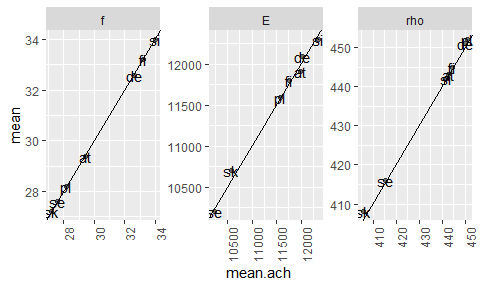Compare achieved coefficients of variation with the defined values. Again, we have undesirable exact values for $$f$$. compare_with_def(dataset_c, ssd_c, 'cov')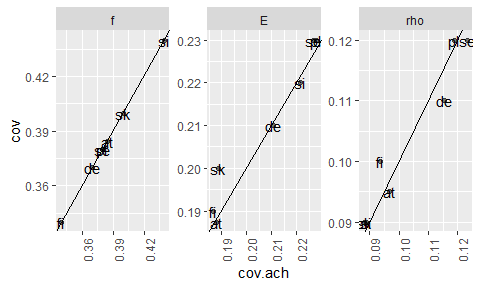## Different subsample sizes ssd_cn <- get_subsample_definitions( country = c(at = 1, de = 3, fi = 1.5, pl = 2, se = 3, si = 1, sk = 1), loadtype = 't' ); dataset_cn <- simulate_dataset( random_seed = 12345, n = 5000, subsets = ssd_cn ); #> Warning in RNGkind("Mersenne-Twister", "Inversion", "Rounding"): non-uniform #> 'Rounding' sampler used summ_fun(dataset_cn); country subsample loadtype n f_mean E_mean rho_mean E_dyn_u_mean ip_f_mean E_dyn_mean ip_rho_mean at at t 400 29.4 (38) 12189 (19) 444 (10) 11320 (18) 30.1 (30) 12815 (17) 460 (9) de de t 1200 32.6 (37) 12102 (21) 451 (11) 11302 (19) 30.2 (33) 12861 (19) 464 (11) fi fi t 600 33.2 (34) 11838 (18) 445 (10) 11116 (18) 29.7 (30) 12600 (17) 459 (9) pl pl t 800 28.2 (38) 11501 (23) 451 (12) 10885 (21) 27.4 (37) 12378 (21) 465 (11) se se t 1200 27.6 (38) 10206 (23) 417 (12) 9769 (22) 23.9 (39) 10989 (22) 432 (11) si si t 400 34.0 (44) 12218 (21) 441 (9) 11326 (19) 30.7 (34) 12801 (19) 457 (9) sk sk t 400 27.2 (40) 10716 (19) 407 (9) 10042 (18) 26.0 (32) 11301 (18) 426 (9) f E rho E_dyn_u ip_f E_dyn ip_rho f 1 0.7386 0.3347 0.6617 0.7733 0.68 0.3249 E 0.7386 1 0.6581 0.9214 0.9231 0.9601 0.6675 rho 0.3347 0.6581 1 0.6852 0.4949 0.7485 0.9523 E_dyn_u 0.6617 0.9214 0.6852 1 0.8715 0.9547 0.6991 ip_f 0.7733 0.9231 0.4949 0.8715 1 0.8985 0.4798 E_dyn 0.68 0.9601 0.7485 0.9547 0.8985 1 0.7673 ip_rho 0.3249 0.6675 0.9523 0.6991 0.4798 0.7673 1 ## Further available options • bending strength simulation • without log-transform • from own data using simbase_covar() # Add simulated values to a dataset For adding simulated values to a dataset, we first need to establish the relationship between these values and some variables in the dataset. In WoodSimulatR, relationships are established in the following way: 1. We determine the covariance matrix and the means of a set of variables, based on some kind of learning dataset. This is done using the function simbase_covar(); the resulting simbase has class “simbase_covar”. 2. We also have the option to establish different relationships for different subsets of the data, e.g. for different countries of origin. This is done by grouping the dataset accordingly before calling simbase_covar(); the resulting simbase has class “simbase_list”. For both these options, it is possible to transform the involved variables. To visualise the result of the simulation, we use scatterplots and define them in the following function: plot_sim_gdp <- function(ds, simb, simulated_vars, ...) { extra_aes <- rlang::enexprs(...); ds <- dplyr::rename(ds, f_ref = f, E_ref = E, rho_ref = rho); if (!any(simulated_vars %in% names(ds))) ds <- simulate_conditionally(data = ds, simbase = simb); ds <- tidyr::pivot_longer( data = ds, cols = tidyselect::any_of(c('f_ref', 'E_ref', 'rho_ref', simulated_vars)), names_to = c('name', '.value'), names_sep = '_' ); ds <- dplyr::mutate( ds, name = factor(name, levels = c('f', 'E', 'rho'), ordered = TRUE) ); simname <- names(ds); simname <- simname[dplyr::cumany(simname == 'name')]; simname <- setdiff(simname, c('name', 'ref')); stopifnot(length(simname) == 1); ggplot(data = ds, mapping = aes(.data[[simname]], ref, !!!extra_aes)) + geom_point(alpha = .2, shape = 20) + geom_abline(slope = 1, intercept = 0, alpha = .5, linetype = 'twodash') + facet_wrap(vars(name), scales = 'free') + theme(axis.text.x = element_text(angle = 90)); } # undebug(plot_sim_gdp) ## simbase_covar without transformation The main approach in WoodSimulatR is to conditionally simulate based on the means and the covariance matrix. As a start, we create basis data for the simulation without applying any transformation. As we later want to add simulated GDP values to a dataset which already contains GDP values, we rename the GDP values for the simbase_covar to some other names not yet present in the target dataset, by suffixing with _siml (for SIMulation with Linear relationships) sb_untransf <- dataset_0 %>% dplyr::rename(f_siml = f, E_siml = E, rho_siml = rho) %>% simbase_covar( variables = c('f_siml', 'E_siml', 'rho_siml', 'ip_f', 'E_dyn', 'ip_rho') ); sb_untransf; #>$label
#>  "n5000_t_cov"
#>
#> $variables #>  "f_siml" "E_siml" "rho_siml" "ip_f" "E_dyn" "ip_rho" #> #>$transforms
#> list()
#>
#> $covar #> f_siml E_siml rho_siml ip_f E_dyn ip_rho #> f_siml 123.48879 17833.53 176.0599 74.39333 16294.87 157.3809 #> E_siml 17833.52632 5350561.53 60442.9207 19384.22862 4936929.13 60112.9581 #> rho_siml 176.05989 60442.92 2277.8973 165.03216 71523.40 2051.2843 #> ip_f 74.39333 19384.23 165.0322 84.82026 18296.48 154.1760 #> E_dyn 16294.87244 4936929.13 71523.3978 18296.48185 5061578.82 71714.5604 #> ip_rho 157.38089 60112.96 2051.2843 154.17601 71714.56 2083.1286 #> #>$means
#>      f_siml      E_siml    rho_siml        ip_f       E_dyn      ip_rho
#>    27.44698 10902.33238   426.68168    25.84706 11646.95452   443.01789
#>
#>  "t"
#>
#> attr(,"class")
#>  "simbase_covar"

Adding the simulated GDP values to a dataset is done by calling simulate_conditionally().

dataset_c_sim <- simulate_conditionally(dataset_c, sb_untransf);
names(dataset_c_sim) %>% pander::pander();

f, E, rho, country, subsample, E_dyn_u, ip_f, E_dyn, ip_rho, loadtype, f_siml, E_siml and rho_siml

For a visual comparison:

plot_sim_gdp(dataset_c_sim, sb_untransf, c('f_siml', 'E_siml', 'rho_siml'));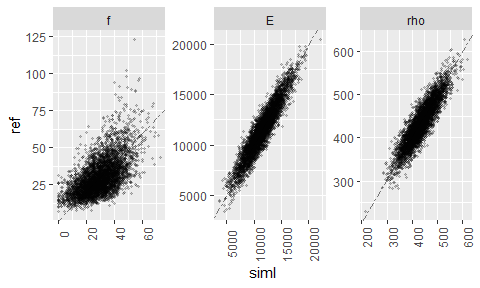This looks good for $$E$$ and $$\rho$$, but wrong in the $$f$$ simulation.

## simbase_covar with log-transformed $$f$$

We might try using transforms to improve the result. For this, we have to pass a list with named entries corresponding to the GDP we want to transform.

The entry itself must be an object of class "trans" (from the package scales). As we want to use a log-transform, the required entry is scales::log_trans().

sb_transf <- dataset_0 %>%
dplyr::rename(f_simt = f, E_simt = E, rho_simt = rho) %>%
simbase_covar(
variables = c('f_simt', 'E_simt', 'rho_simt', 'ip_f', 'E_dyn', 'ip_rho'),
transforms = list(f_simt = scales::log_trans())
);
dataset_c_sim <- simulate_conditionally(dataset_c_sim, sb_transf);
plot_sim_gdp(dataset_c_sim, sb_transf, c('f_simt', 'E_simt', 'rho_simt'));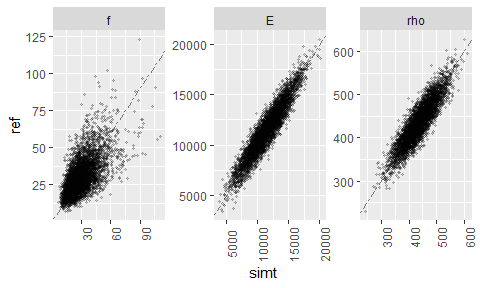Now, this looks much better (which is no surprise, as dataset_c itself has been simulated with lognormal $$f$$).

## simbase_covar with log-transformed $$f$$ and derived on a grouped dataset

If we group the reference dataset (dataset_0), e.g. by country, we get an object of class “simbase_list” with separate simbases for each group (technically, this is a tibble with the grouping variables and an extra column .simbase which contains several objects of class “simbase_covar”).

sb_group <- dataset_0 %>%
dplyr::group_by(country) %>%
dplyr::rename(f_simg = f, E_simg = E, rho_simg = rho) %>%
simbase_covar(
variables = c('f_simg', 'E_simg', 'rho_simg', 'ip_f', 'E_dyn', 'ip_rho'),
transforms = list(f_simg = scales::log_trans())
);

sb_group
#> # A tibble: 4 x 2
#>   country .simbase
#>   <chr>   <list>
#> 1 C1      <smbs_cvr>
#> 2 C2      <smbs_cvr>
#> 3 C3      <smbs_cvr>
#> 4 C4      <smbs_cvr>

If we add variables to a dataset using such a “simbase_list”, it is required that all grouping variables stored in the “simbase_list” object are also available in this dataset.

In our case: the dataset must contain the variable “country”. Values of “country” which do not also exist in our “simbase” object will result in NA values for the variables to be simulated.

Therefore, we add the variables in this case not to the dataset dataset_c (which has different values for “country”) but to the dataset_0 itself.

dataset_0_sim <- simulate_conditionally(dataset_0, sb_group);
plot_sim_gdp(dataset_0_sim, sb_group, c('f_simg', 'E_simg', 'rho_simg'), colour=country);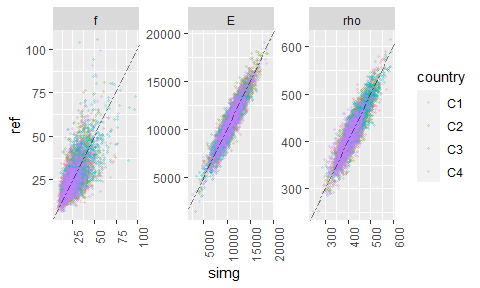# Simulate a whole dataset, based on a simbase_list object

Simbase objects of class “simbase_list” can also be used for simulating an entire dataset, as long as the “simbase_list” only has the grouping variable(s) “country” and/or “subsample”, and as long as the value combinations in “country”/“subsample” match those given in the “subsets” argument to the function simulate_dataset.

To demonstrate, we calculate a “simbase_list” based on the dataset_c created above. Here, we do not rename any of the variables.

sb_group_c <- dataset_c %>%
dplyr::group_by(country) %>%
simbase_covar(
variables = c('f', 'E', 'rho', 'ip_f', 'E_dyn', 'ip_rho'),
transforms = list(f = scales::log_trans())
);

sb_group_c
#> # A tibble: 7 x 2
#>   country .simbase
#>   <chr>   <list>
#> 1 at      <smbs_cvr>
#> 2 de      <smbs_cvr>
#> 3 fi      <smbs_cvr>
#> 4 pl      <smbs_cvr>
#> 5 se      <smbs_cvr>
#> 6 si      <smbs_cvr>
#> 7 sk      <smbs_cvr>

This “simbase_list” is now used as input to simulate_dataset with the subset definitions used previously (ssd_cn).

dataset_cn2 <- simulate_dataset(
random_seed = 12345,
n = 5000,
subsets = ssd_cn,
simbase = sb_group_c
);
#> Warning in RNGkind("Mersenne-Twister", "Inversion", "Rounding"): non-uniform
#> 'Rounding' sampler used

summ_fun(dataset_cn2);
country subsample loadtype n f_mean E_mean rho_mean ip_f_mean E_dyn_mean ip_rho_mean
at at t 400 29.4 (38) 12190 (19) 444 (10) 29.9 (30) 12844 (17) 460 (9)
de de t 1200 32.6 (37) 12122 (20) 450 (11) 30.2 (31) 12891 (19) 465 (11)
fi fi t 600 33.2 (34) 11903 (19) 445 (10) 29.7 (29) 12698 (18) 459 (10)
pl pl t 800 28.2 (38) 11620 (24) 451 (12) 27.8 (39) 12459 (22) 465 (11)
se se t 1200 27.6 (38) 10196 (22) 417 (12) 23.9 (37) 10983 (20) 433 (11)
si si t 400 34.0 (44) 12466 (22) 442 (9) 31.6 (34) 13100 (19) 460 (8)
sk sk t 400 27.2 (40) 10563 (21) 406 (9) 25.5 (36) 11245 (19) 426 (9)
f E rho ip_f E_dyn ip_rho
f 1 0.7467 0.3238 0.7827 0.6814 0.3103
E 0.7467 1 0.6582 0.9221 0.9601 0.6651
rho 0.3238 0.6582 1 0.4939 0.7474 0.9515
ip_f 0.7827 0.9221 0.4939 1 0.8992 0.4804
E_dyn 0.6814 0.9601 0.7474 0.8992 1 0.767
ip_rho 0.3103 0.6651 0.9515 0.4804 0.767 1

# Conclusions

The package WoodSimulatR has functions for simulating entire datasets of sawn timber properties, both based on internal definitions and on externally supplied base data.

WoodSimulatR also has functions for adding simulated grade determining properties (or other properties) to a given dataset, based on a covariance matrix approach.

The functions for adding simulated variables are suitable for all kinds of datasets, if one calculates an appropriate simbase_covar object oneself, by a call to simbase_covar using reference data.

The simulation methods also support variable transformations to accommodate non-normally distributed variables.